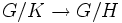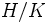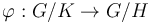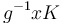# Index is multiplicative

(diff) ← Older revision | Latest revision (diff) | Newer revision → (diff)
Jump to: navigation, search
This article gives the statement, and possibly proof, of a subgroup property (i.e., subgroup of finite index) satisfying a subgroup metaproperty (i.e., transitive subgroup property)
View all subgroup metaproperty satisfactions | View all subgroup metaproperty dissatisfactions |Get help on looking up metaproperty (dis)satisfactions for subgroup properties
Get more facts about subgroup of finite index |Get facts that use property satisfaction of subgroup of finite index | Get facts that use property satisfaction of subgroup of finite index|Get more facts about transitive subgroup property

## Statement

### Set-theoretic version

Suppose$K \le H \le G$ are groups. Then, we have a natural surjective map between the left coset spaces:$G/K \to G/H$

with the property that the inverse image of each point has size equals to the size of the left coset space$H/K$.

### Numerical version

Suppose$K \le H \le G$ are groups such that the indices$[G:H]$ and$[H:K]$ are finite. Then, we have:$[G:K] = [G:H][H:K]$.

In particular, if$K$ has finite index in$H$ and$H$ has finite index in$G$, then$K$ has finite index in$G$.

Note also that if$[G:K]$ is finite, then so are the other two indices, and we thus get:$[G:K] = [G:H][H:K]$.

Note that the statement makes sense even for infinite groups, if we interpret the cardinalities of the coset spaces as infinite cardinals and do the multiplication using the rules for product of cardinals.

## Related facts

• Lagrange's theorem: Lagrange's theorem is a special case of this where$K$ is the trivial group.
• Third isomorphism theorem: The third isomorphism theorem is a stronger version of the statement where both subgroups are normal in the whole group. In this case, the surjective map from$G/K$ to$G/H$ is a homomorphism and the kernel is$H/K$.

## Facts used

1. Subgroup containment implies coset containment: If$K \le H \le G$ ,then every left coset of$K$ is contained in a unique left coset of$H$.

## Proof

### Proof of the set-theoretic version

Given: Groups$K \le H \le G$.

To prove: There is a surjective map from$G/K$ to$G/H$ where the inverse image of every point has size equal to the size of$H/K$.

Proof: Define$\varphi$ as the map sending a coset of$K$ to the unique coset of$H$ containing it (fact (1)). In other words:$\varphi(gK) = gH$.$\varphi$ is a well-defined map from$G/K$ to$G/H$ by Fact (1).

#### Surjectivity of the map$\varphi$ is surjective, since for any coset$gH$ of$H$ in$G$,$gH = \varphi(gK)$.

#### Size of the inverse images

To prove: Consider the map$\varphi: G/K \to G/H$. Choose$g \in G$ and consider the left coset$gH$. Then, the subset of$G/K$ comprising left cosets contained in$gH$ (i.e., left cosets that map to$gH$ under$\varphi$) can be put in bijection with$H/K$.

Proof: We explicitly construct such a bijection$\psi$ based on the choice of$g$, defined as:$\psi(xK) = g^{-1}xK$

Note that:

• This map$\psi$ is well-defined, because if$x$ is replaced by$xk, k \in K$, the left cosets$g^{-1}xK$ and$g^{-1}xkK$ are the same.
• The map$\psi$ sends left cosets of$K$ in$gH$ to left cosets of$K$ in$H$, because since$x \in gH$,$g^{-1}x \in H$.
• The map is injective, because it comes from a left multiplication on cosets.
• The map is surjective, because any left coset$hK$ of$K$ in$H$ arises as the image of the left coset$ghK$, which lies in$gH$.

Thus,$\psi$ is a bijection between the left cosets of$K$ in$gH$ and the left cosets of$K$ in$H$. Thus, the number of left cosets of$K$ in$gH$ equals the size of the left coset space$H/K$.

### Proof of the numerical version

The set-theoretic version shows that$G/K$ is the disjoint union of$|G/H|$ sets, each of size$|H/K|$. This yields:$|G/K| = |G/H||H/K|$.

By the definition of index of a subgroup, this yields:$[G:K] = [G:H][H:K]$.